# Surface Area and Volume : Exercise - 13.4 (Mathematics NCERT Class 10th)

Use $\pi = {{22} \over 7}$ unless stated otherwise.

Q.1     A drinking glass is in the  shape of a frustum of a cone of height  14 cm. The  diameters of its two circular ends are 4 cm and 2 cm. Find  the capacity of the glass.
Sol.

Capacity of the glass,
$V = {{\pi \times h} \over 3} \times \left( {{R^2} + {r^2} + Rr} \right)$
Here, R = 2 cm , r = 1 cm , h = 14 cm
Therefore     $V = {{{{22} \over 7}} \over 3} \times \left( {{2^2} + {1^2} + 2 \times 1} \right)$
$= {{44} \over 3} \times \left( {4 + 1 + 2} \right)$
$= {{44} \over 3} \times 7 = {{308} \over 3}102{2 \over 3}c{m^3}$

Q.2     The slant height  of a frustum of a cone is 4 cm and the perimeters (circumference) of its circular ends are 18 cm and 6 cm. Find  the curved surface area  of the frustum.
Sol.

Slant height ,l = 4 cm (Given)
$2\pi {r_1} = 6$        $\Rightarrow$    $\pi {r_1} = 3$
and   $2\pi {r_2} = 18$       $\Rightarrow$    $\pi {r_2} = 9$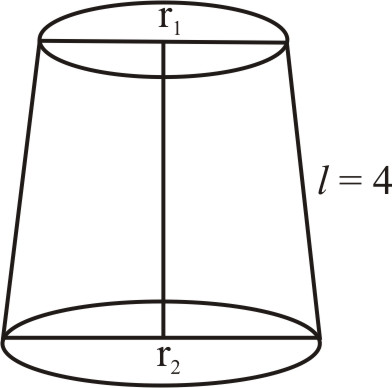Curved surface of the  frustum
$= \left( {\pi {r_1} + \pi {r_2}} \right)\ell$
$= \left( {3 + 9} \right) \times 4\,c{m^2}$
$= 12 \times 4\,c{m^2}$
$= 48\,c{m^2}$

Q.3      A fez, the  (cap) used by the Turks, is  shaped like the frustum of a cone (see figure). If its radius on the open side is 10 cm, radius at the upper base is 4 cmand its slant height is 15 cm, find the area of material used for  making it.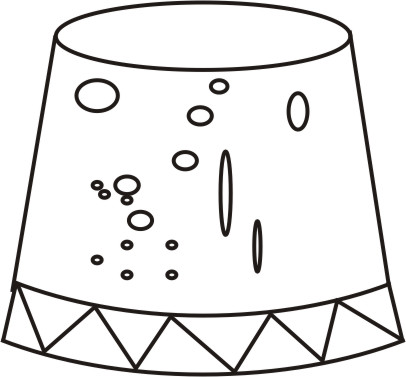Sol.

Here  R = 10 cm, r = 4 cm and l = 15 cm
Area of the material used for making the feza = Surface area of frustum
+  The  surface of top circular section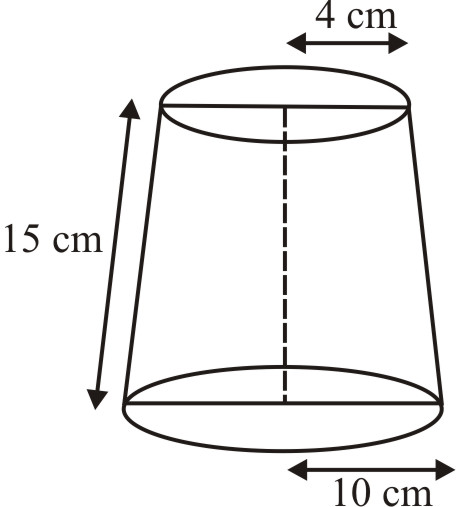$= \pi \left( {R + r} \right)\ell + \pi {r^2}$
$= {{22} \over 7}\left( {10 + 4} \right)15 + {{22} \over 7} \times 4 \times 4$
$= \left( {{{22} \over 7} \times 14 \times 15 + {{352} \over 7}} \right)c{m^2}$
$= {{4620 + 352} \over 7}c{m^2} = {{4972} \over 7}c{m^2}$
$= 710{2 \over 7}c{m^2}$

Q.4      A container  opened from  the top and made up of a metal  sheet, is in the form of a frustum of a cone of height 16 cm with radii of its lower and upper ends as 8 cm and 20 cm, respectively. Find the cost of milk which can completely fill the container, at the rate of Rs 20 per litre. Also  find the  cost of metal sheet used to make the container, if it costs Rs 8 per 100 $c{m^2}$. (Take $\pi = 3.14$).
Sol.

Here R = 20 cm, r = 8 cm and h = 16 cm Capacity of the container
= Volume of the frustum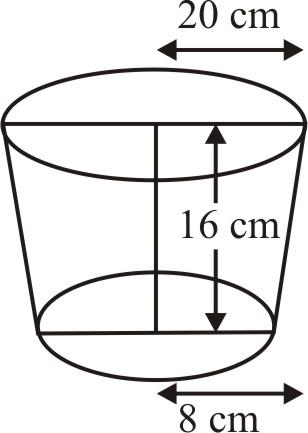$= {1 \over 3}\pi h\left( {{R^2} + {r^2} + Rr} \right)$
$= {1 \over 3} \times 3.14 \times 16\left( {{{20}^2} + {8^2} + 20 \times 8} \right)c{m^3}$
$= {{50.24} \over 3} \times \left( {400 + 64 + 160} \right)c{m^3}$
$= {{50.24} \over 3} \times 624\,c{m^3} = 50.24 \times 208\,c{m^3}$
$= {{10449.92} \over {1000}}litres$
Cost of milk @ Rs 20 per litre
$= Rs\left( {20 \times {{10449.92} \over {1000}}} \right)$
$= Rs\,\,208.99 \approx Rs\,209$
To find the slant height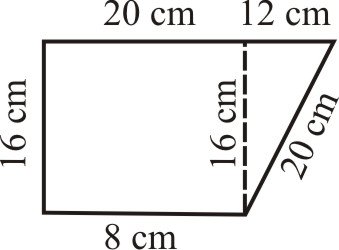$\ell = \sqrt {{{16}^2} + {{12}^2}}$
$= \sqrt {256 + 144}$
$\sqrt {400} = 20\,cm$
$= \pi \left( {R + r} \right)\ell$
$= {{22} \over 7} \times \left( {20 + 8} \right) \times 20\,c{m^2}$
$= {{22} \over 7} \times 28 \times 20\,c{m^2} = 1758.4\,c{m^2}$
and area of the bottom
$= \pi {r^2} = {{22} \over 7} \times {\left( 8 \right)^2} = {{22} \over 7} \times 64 = 200.96\,c{m^2}$
Therefore  Total area of metal required
= 1758.4 $c{m^2} + 200.96\,c{m^2} = 1959.36\,c{m^2}$
Cost of metal sheet used to manufacture the container @ Rs 8 per $100\,c{m^2}$
$= Rs\left( {{8 \over {100}} \times 1959.36} \right) = Rs\,156.75$

Q.5     A metallic right  circular cone 20 cm high  and whose vertical angle is 60º is cut  into two parts at the middle of its height by a plane parallel to its base. If the frustum so obtained be drawn into wire of diameter ${1 \over {16}}cm$, find the length of the wire.
Sol.

Let ABC be the metallic cone, DECB is the required frustum.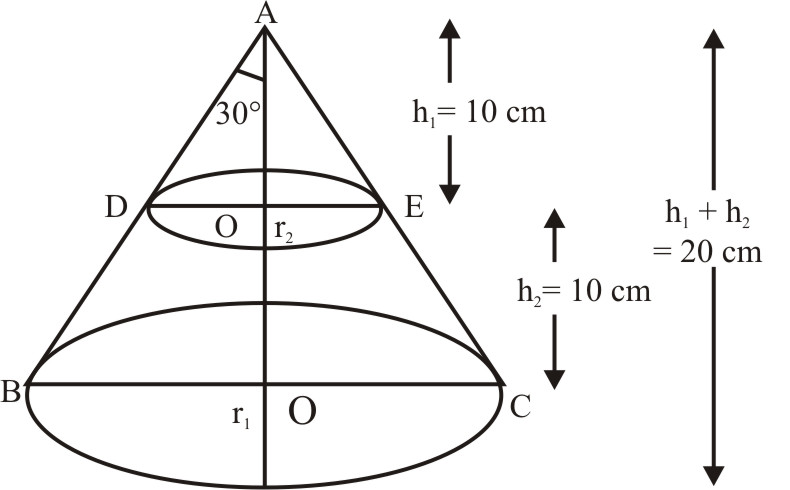Let the two  radii of the frustum be $DO' = {r_2}\,and\,BO = {r_1}$
$DO' = {r_2}\,and\,BO = {r_1}$
From the $\Delta$s ADO' and ABO,
${r_2} = {h_1}\,\tan \,{30^o} = 10 \times {1 \over {\sqrt 3 }}$
${r_1} = \left( {{h_1} + {h_2}} \right)\tan \,{30^o}$
$= 20 \times {1 \over {\sqrt 3 }}$
Volume of the  frustum DBCE
$= {{\pi {h_2}} \over 3}\left( {{r_1}^2 + {r_1}{r_2} + {r_2}^2} \right)$
$= {{\pi {h_2}} \over 3} \times \left[ {{{400} \over 3} + {{200} \over 3} + {{100} \over 3}} \right]$
${{\pi� \times 10} \over 3} \times {{700} \over 3}$
Volume of the wire  of length l and  diameter D
$= \pi {\left( {{D \over 2}} \right)^2} \times \ell = {{\pi {D^2}} \over 4} \times \ell$        $\left[ {V = \pi {r^2}h} \right]$
Therefore  Volume of the frustum = Volume  of the wire drawn from it
$\Rightarrow$    ${{\pi \times 10} \over 3} \times {{700} \over 3} = {{\pi {D^2}} \over 4} \times \ell$        $\left[ {\sin ce\,D = {1 \over {16}}} \right]$
$\Rightarrow$    $\ell = {{10 \times 700 \times 4} \over {3 \times 3}} \times 16 \times 16$
= 7964.44 m